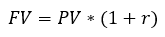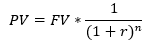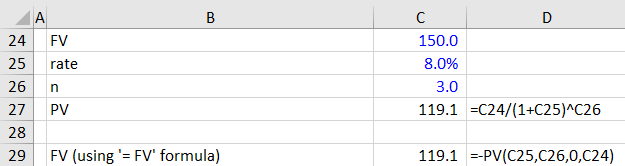## What is Present Value and Future Value?

A common question that I come across in class relates to the difference between present value (PV) and future value (FV). Most people intuitively understand that it is better to have \$100 today than it is to wait a year to get \$100. They often find it difficult to articulate why though so let’s look at some of the math involved.

If you deposit some money with the bank you would expect it to earn interest (even though interest rates are very low at the moment!) and your deposit would therefore be worth more in one year’s time:PV = initial deposit

FV = initial deposit plus total interest earned

r = interest rate

## Example Calculation

So if you deposit \$100 at an interest rate of 5%, you would expect to have \$105 in one year’s time. But how much would you expect to have in two years? It would not simply be \$110 because you would have actually invested \$105 for the second year. Let’s expand the equation to incorporate investments of more than a year:Where n = time in years (assuming interest is paid annually).

This process is called ‘compounding’ and is used to calculate compound interest. The opposite process is called ‘discounting’ which is used extensively in discounted cash flow (DCF) valuation when we want to estimate the present value of future cash flows. Rearranging the previous equation:Let’s say that you know you are going to need \$150 in three years time to buy something special and you have found a special term deposit at your bank that is paying an annual interest rate of 8%. How much do you need to invest today in order to have \$150 in three years?

We know that FV = \$150, r = 8% and n = 3, so we have everything we need to calculate the present value of our investment which turns out to be about \$119.07.

There are some very useful functions in Excel that can help with this. For example, the ‘=PV()’ function will calculate the present value providing you know the rate, number of periods nper and future value FV (the PMT value should be zero in this case as there are no interim cash flows).